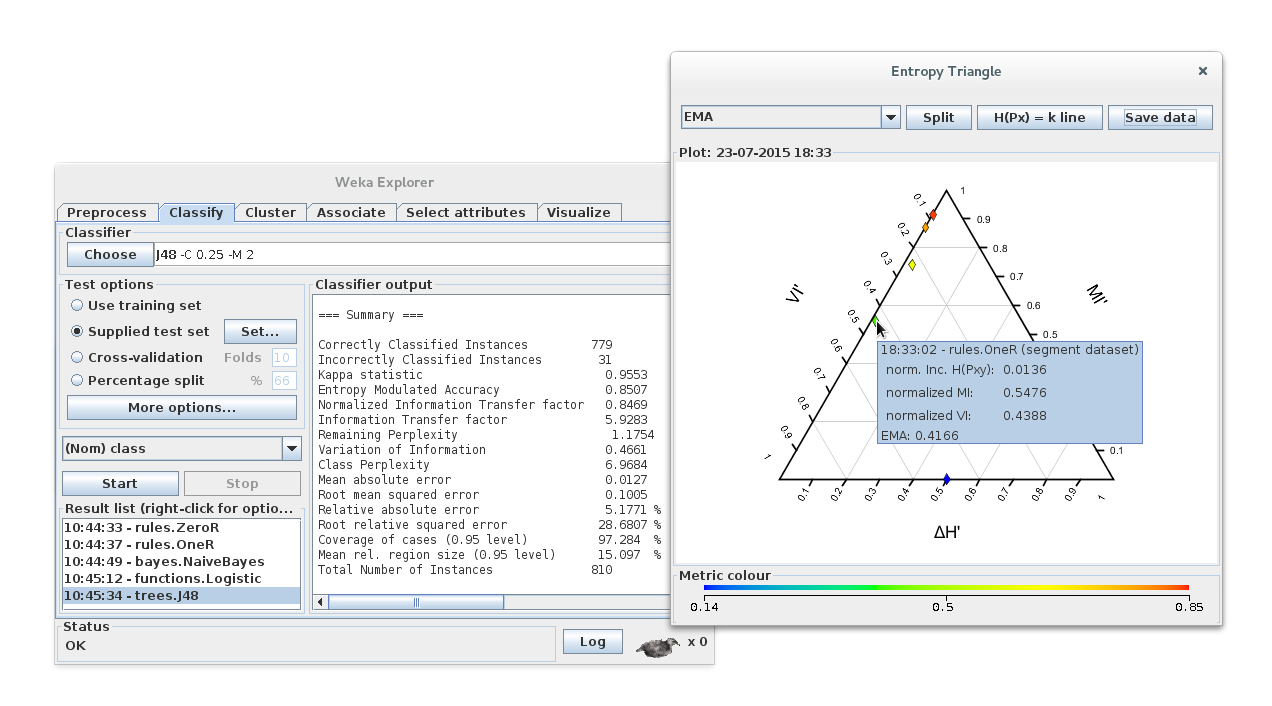# Entropy Triangle Weka package

A set of information-theoretic tools for the assessment of multi-class classifiers in Weka## Features

• Visualization Plugin with an exploratory data analysis method for an easy, but complete and reliable comparison of classifiers performance.

The Entropy Triangle is based on a balance equation of entropies. Represents normalized values of the variation of information, the mutual information, and the increment of entropy from the uniform distribution in a ternary plot. This lets you analyze at a glance different scenarios without loss of information.

• Panel with colorbar (or labels) for the chosen metric (or classifier/dataset information)
• Tooltips with detailed information hovering graph elements
• Delete elements of the graph with a right-click context menu
• Save and load graph data in arff format
• Export data to csv or json
• Print the graph with Ctrl+Shft+Alt+Left Mouse Click
• New evaluation metrics for the standard output of Weka.

• Entropy Modulated Accuracy (EMA)

The EMA is a pessimistic estimate of the accuracy with the influence of the input distribution factored out. It is defined mathematically as the inverse of the remaining perplexity after the learning process

$$EMA = \frac{1}{PP_{X|Y}} = \frac{1}{2^{H_{X|Y}}}$$

• Normalized Information Transfer factor (NIT factor).

A measure of how efficient is the transmission of information from the input to the output of the classifier

$$NIT_{factor} = \frac{\mu_{XY}}{k}$$ where k is the number of classes.

• Class perplexity

The class perplexity can be interpreted as the number of effective classes at the input of the classifier, due to the likelihood of the input distribution

$$PP_X = 2^{H_X}$$

• Remaining perplexity

The Remaining Perplexity measures the residual difficulty after the learning process $$PP_{X|Y} = 2^{H_{X|Y}}$$

• Information Transfer factor

The Information Transfer factor measures the variation in difficulty due to the mutual information between the input and the output distributions of the classifier. $$\mu_{XY} = 2^{MI_{XY}}$$

• Variation of information $$VI = H_{X|Y} + H_{Y|X}$$

## Theoretical background

This package is an implementation of the work of these papers: